Function Repository Resource:

# DarbouxVector

Compute the Darboux vector field of a curve

Contributed by: Wolfram Staff (original content by Alfred Gray)
 ResourceFunction["DarbouxVector"][α,t] computes the Darboux vector field of a curve.

## Details and Options

The Darboux vector of a space curve with unit speed is the angular velocity vector of the Frenet frame.

## Examples

### Basic Examples (3)

Define and plot a helix:

 In:=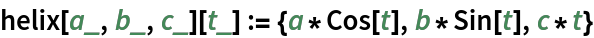In:=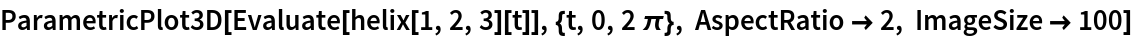Out=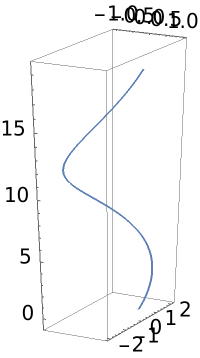Compute the Darboux vector of the helix and plot its components:

 In:=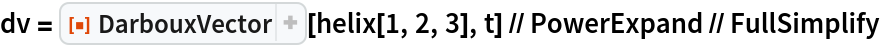Out=In:=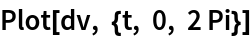Out=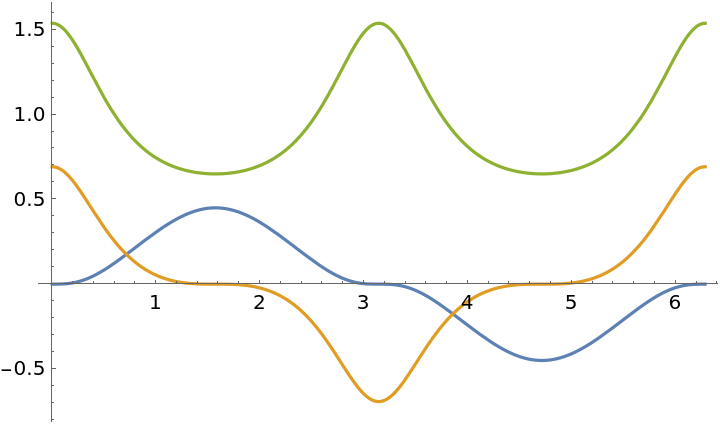Plot the Darboux vector in space:

 In:=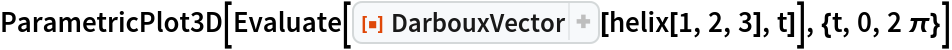Out=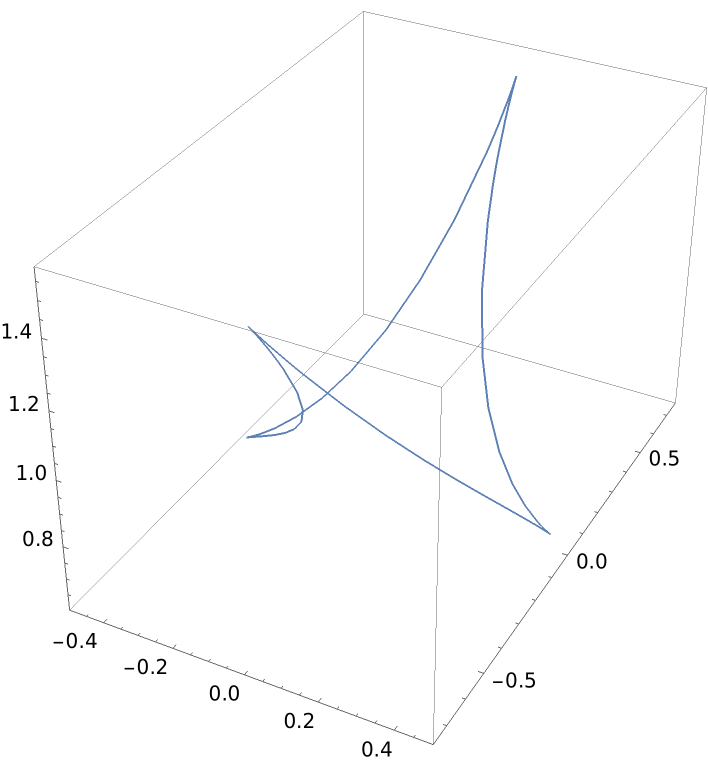### Properties and Relations (1)

The Darboux vector can be expressed in terms of the Frenet-Serret system of the curve:

 In:=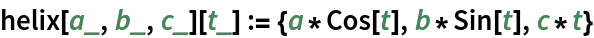In:=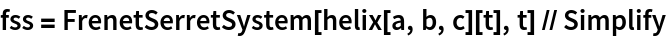Out=In:=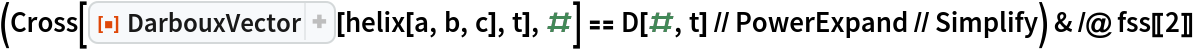Out=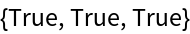Enrique Zeleny

## Version History

• 1.0.0 – 20 February 2020